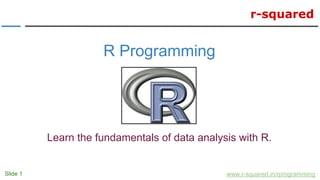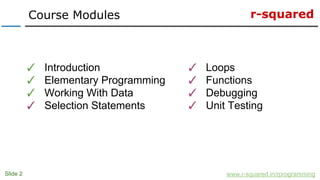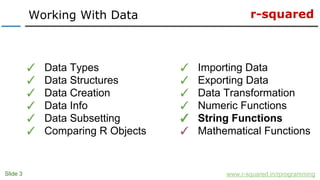Se está descargando tu SlideShare. ×

# R Programming: Learn To Manipulate Strings In R

Anuncio
Anuncio
Anuncio
Anuncio
Anuncio
Anuncio
Anuncio
Anuncio
Anuncio
Anuncio
AnuncioCargando en…3
×

1 de 41 Anuncio

# R Programming: Learn To Manipulate Strings In R

Learn to manipulate strings in R using the built in R functions. This tutorial is part of the Working With Data module of the R Programming Course offered by r-squared.

Learn to manipulate strings in R using the built in R functions. This tutorial is part of the Working With Data module of the R Programming Course offered by r-squared.

Anuncio
Anuncio

### R Programming: Learn To Manipulate Strings In R

1. 1. r-squared Slide 1 www.r-squared.in/rprogramming R Programming Learn the fundamentals of data analysis with R.
2. 2. r-squared Slide 2 Course Modules www.r-squared.in/rprogramming ✓ Introduction ✓ Elementary Programming ✓ Working With Data ✓ Selection Statements ✓ Loops ✓ Functions ✓ Debugging ✓ Unit Testing
3. 3. r-squared Slide 3 Working With Data www.r-squared.in/rprogramming ✓ Data Types ✓ Data Structures ✓ Data Creation ✓ Data Info ✓ Data Subsetting ✓ Comparing R Objects ✓ Importing Data ✓ Exporting Data ✓ Data Transformation ✓ Numeric Functions ✓ String Functions ✓ Mathematical Functions
4. 4. r-squared In this unit, we will explore string manipulation in R using the following functions: Slide 4 String Functions www.r-squared.in/rprogramming ● match() ● char.expand() ● grep() ● grepl() ● sub() ● substr() ● substring() ● strsplit() ● strtrim() ● chartr() ● tolower() ● toupper() ● toString() ● nchar() ● nzchar() ● noquote() ● pmatch() ● charmatch()
5. 5. r-squared Slide 5 chartr() www.r-squared.in/rprogramming Description chartr() replaces characters in a string. Syntax chartr(old, new, x) Returns String with replaced characters Documentation help(chartr)
6. 6. r-squared Slide 6 chartr() www.r-squared.in/rprogramming Examples > # example 1 > name <- "Jovial Mann" > chartr("J", "K", name)  "Kovial Mann" > # example 2 > name <- "Jovial Mann" > chartr("a", "e", name)  "Joviel Menn"
7. 7. r-squared Slide 7 tolower()/toupper() www.r-squared.in/rprogramming Description tolower() translates characters from upper to lower case. toupper() translates characters from lower to upper case. Syntax tolower(x) toupper(x Returns String translated to lower or upper case Documentation help(tolower) help(toupper)
8. 8. r-squared Slide 8 tolower()/toupper() www.r-squared.in/rprogramming Examples > # example 1 > name <- "Jovial Mann" > tolower(name)  "jovial mann" > # example 2 > name <- "Jovial Mann" > tolower(name)  "jovial mann" > toupper(name)  "JOVIAL MANN"
9. 9. r-squared Slide 9 toString() www.r-squared.in/rprogramming Description toString() converts an R object to character string. Syntax toString(x) Returns Character vector of length 1 Documentation help(toString)
10. 10. r-squared Slide 10 toString() www.r-squared.in/rprogramming Examples > example 1 > x <- 100 > toString(x)  "100" > name <- c("Jovial", "Mann") > name  "Jovial" "Mann" > toString(name)  "Jovial, Mann" > toString(name, width = 8)  "Jovi...." > toString(name, width = 12)  "Jovial, Mann" > toString(name, width = 6)  "Jo...."
11. 11. r-squared Slide 11 nchar() www.r-squared.in/rprogramming Description nchar() returns the size of character strings. It takes a character vector as argument and returns a vector that contains the size of the elements of the argument. Syntax nchar(character vector) Returns Integer vector Documentation help(nchar)
12. 12. r-squared Slide 12 nchar() www.r-squared.in/rprogramming Examples > # example 1 > name <- c("Jovial", "Mann") > nchar(name)  6 4 > first_name <- "Jovial" > nchar("first_name")  10 > last_name <- "Mann" > nchar(last_name)  4
13. 13. r-squared Slide 13 nzchar() www.r-squared.in/rprogramming Description nzchar() tests whether elements of a character vector are non-empty strings. Syntax nzchar(character vector) Returns Logical vector Documentation help(nzchar)
14. 14. r-squared Slide 14 nzchar() www.r-squared.in/rprogramming Examples > # example 1 > name <- c("Jovial", "Mann") > nzchar(name)  TRUE TRUE > # example 2 > details <- c("Jovial", "") > nzchar(details)  TRUE FALSE
15. 15. r-squared Slide 15 noquote() www.r-squared.in/rprogramming Description noquote() returns character strings without quotes. Syntax noquote(object) Returns Character strings without quotes Documentation help(noquote)
16. 16. r-squared Slide 16 noquote() www.r-squared.in/rprogramming Examples > # example 1 > letters  "a" "b" "c" "d" "e" "f" "g" "h" "i" "j" "k" "l" "m" "n" "o" "p" "q" "r" "s" "t" "u" "v"  "w" "x" "y" "z" > nql <- noquote(letters) > nql  a b c d e f g h i j k l m n o p q r s t u v w x y z
17. 17. r-squared Slide 17 pmatch() www.r-squared.in/rprogramming Description pmatch() performs partial string matching. It takes two arguments and seeks matches for the elements of the first argument in the second. Syntax pmatch(x, table) Returns Integer vector indicating index of elements that have a match Documentation help(pmatch)
18. 18. r-squared Slide 18 pmatch() www.r-squared.in/rprogramming Examples > # example > pmatch("Jov", c("Jovial", "Jovathon", "John"))  NA > pmatch("Jovi", c("Jovial", "Jovathon", "John"))  1 > pmatch("m", c("mean", "median", "mode"))  NA > pmatch("med", c("mean", "median", "mode"))  2
19. 19. r-squared Slide 19 charmatch() www.r-squared.in/rprogramming Description charmatch() performs partial string matching. It takes two arguments and seeks matches for the elements of the first argument in the second. Syntax charmatch(x, table) Returns Integer vector indicating index of elements that have a match Documentation help(charmatch)
20. 20. r-squared Slide 20 charmatch() www.r-squared.in/rprogramming Examples > # example 1 > charmatch("Jov", c("Jovial", "Jovathon", "John"))  0 > charmatch("Jovi", c("Jovial", "Jovathon", "John"))  1 > charmatch("m", c("mean", "median", "mode"))  0 > charmatch("med", c("mean", "median", "mode"))  2
21. 21. r-squared Slide 21 match() www.r-squared.in/rprogramming Description match() takes 2 arguments and returns the index of the character element in the second argument that matches the first one. Syntax match(x, table) Returns Integer vector of characters that match Documentation help(match)
22. 22. r-squared Slide 22 match() www.r-squared.in/rprogramming Examples > # example 1 > x <- sample(1:10) > x  9 8 3 7 1 5 10 2 6 4 > match(5, x)  6 > # example 2 > x <- sample(letters) > x  "t" "e" "i" "b" "h" "g" "c" "q" "u" "s" "r" "z" "j" "v" "y" "k" "l" "w" "p" "m" "d" "a"  "f" "x" "o" "n" > match("a", x)  22
23. 23. r-squared Slide 23 char.expand() www.r-squared.in/rprogramming Description char.expand() takes two arguments and seeks match for the first argument in the elements of its second argument and returns the element if a match is found. Syntax char.expand(input, target) Returns Element in target that is a match for input. Documentation help(char.expand)
24. 24. r-squared Slide 24 char.expand() www.r-squared.in/rprogramming Examples > # example 1 > loc <- c("mean", "median", "mode") > char.expand("me", loc, nomatch = stop("no match")) character(0) > char.expand("mo", loc)  "mode" > char.expand("med", loc)  "median"
25. 25. r-squared Slide 25 grep() www.r-squared.in/rprogramming Description grep() takes two arguments and seeks match for the first argument in the elements of its second argument and returns the index of the elements that match. Syntax grep(pattern, character vector) Returns Integer vector indicating the index of elements in the character vector that match the pattern Documentation help(grep)
26. 26. r-squared Slide 26 grep() www.r-squared.in/rprogramming Examples > # example 1 > grep("a", c("mean", "median", "mode"))  1 2 > grep("d", c("mean", "median", "mode"))  2 3 > grep("me", c("mean", "median", "mode"))  1 2 > grep("od", c("mean", "median", "mode"))  3
27. 27. r-squared Slide 27 grepl() www.r-squared.in/rprogramming Description grepl() takes two arguments and seeks match for the first argument in the elements of its second argument and returns a logical vector if there is a match Syntax grepl(pattern, character vector) Returns Logical vector Documentation help(grepl)
28. 28. r-squared Slide 28 grepl() www.r-squared.in/rprogramming Examples > # example 1 > grepl("a", c("mean", "median", "mode"))  TRUE TRUE FALSE > grepl("d", c("mean", "median", "mode"))  FALSE TRUE TRUE > grepl("me", c("mean", "median", "mode"))  TRUE TRUE FALSE > grepl("od", c("mean", "median", "mode"))#  FALSE FALSE TRUE
29. 29. r-squared Slide 29 sub() www.r-squared.in/rprogramming Description sub() takes three arguments and seeks match for the first argument in the elements of its third argument and replaces them with the second argument. Syntax sub(pattern, replacement, character vector) Returns Character vector with replaced string Documentation help(sub)
30. 30. r-squared Slide 30 sub() www.r-squared.in/rprogramming Examples > # example 1 > str <- "Good Morning" > sub("Go", "Mo", str)  "Mood Morning" > # example 2 > str <- c("mean", "median", "mode" ) > sub("m", "k", str)  "kean" "kedian" "kode"
31. 31. r-squared Slide 31 substr() www.r-squared.in/rprogramming Description substr() extracts or replaces substring in a character vector. Syntax substr(character vector, start, stop) Returns Substring or character vector with replaced substring Documentation help(substr)
32. 32. r-squared Slide 32 substr() www.r-squared.in/rprogramming Examples > # example 1 > substr("abcdef", 2, 4)  "bcd" > # example 2 > substr("abcdef", 2, 4)  "bcd" > substr(rep("abcdef", 4), 1:4, 4:5)  "abcd" "bcde" "cd" "de" > x <- c("asfef", "qwerty", "yuiop[", "b", "stuff.blah.yech") > substr(x, 2, 5)  "sfef" "wert" "uiop" "" "tuff"
33. 33. r-squared Slide 33 substring() www.r-squared.in/rprogramming Description substring() extracts or replaces substring in a character vector. Syntax substr(character vector, first, last) Returns Substring or character vector with replaced substring Documentation help(substring)
34. 34. r-squared Slide 34 substring() www.r-squared.in/rprogramming Examples > # example 1 > substring("abcdef", 1, 1:6)  "a" "ab" "abc" "abcd" "abcde" "abcdef" > x <- c("asfef", "qwerty", "yuiop[", "b", "stuff.blah.yech") > substring(x, 2, 4:6)  "sfe" "wert" "uiop[" "" "tuff" > substring(x, 2) <- c("..", "+++") > x  "a..ef" "q+++ty" "y..op[" "b" "s..ff.blah.yech"
35. 35. r-squared Slide 35 strsplit() www.r-squared.in/rprogramming Description strsplit() takes two arguments and splits the elements of the first argument at the index position of the second argument if the characters in the first argument match the secon argument. Syntax strsplit(character vector, split) Returns List of elements in the first argument split by the second argument. Documentation help(strsplit)
36. 36. r-squared Slide 36 strsplit() www.r-squared.in/rprogramming Examples > # example 1 > x <- c(as = "asfef", qu = "qwerty", "yuiop[", "b", "stuff.blah.yech") > x as qu "asfef" "qwerty" "yuiop[" "b" "stuff.blah.yech" > strsplit(x, "e") \$as  "asf" "f" \$qu  "qw" "rty" []  "yuiop[" []  "b" []  "stuff.blah.y" "ch"
37. 37. r-squared Slide 37 strsplit() www.r-squared.in/rprogramming Examples > # example 2 > y <- c("India", "America", "Australia", "China", "Russia", "Britain") > strsplit(y, "i") []  "Ind" "a" []  "Amer" "ca" []  "Austral" "a" []  "Ch" "na" []  "Russ" "a" []  "Br" "ta" "n"
38. 38. r-squared Slide 38 strtrim() www.r-squared.in/rprogramming Description strtrim() takes two arguments and trims the first argument at the index mentioned in the second argument. Syntax strtrim(character vector, index) Returns Trimmed character vector Documentation help(strtrim)
39. 39. r-squared Slide 39 strtrim() www.r-squared.in/rprogramming Examples > # example 1 > name  "Jovial" "Mann" > strtrim(name, 4)  "Jovi" "Mann" > # example 2 > alphabets <- "aksjdnfkasdjhfkjasdkfalkdsfnkajdsnfkjadn" > strtrim(alphabets, 10)  "aksjdnfkas"
40. 40. r-squared In the next unit, we will explore the following mathematical functions: Slide 40 Next Steps... www.r-squared.in/rprogramming ● abs() ● round() ● ceiling() ● floor() ● trunc() ● signif() ● jitter() ● format() ● formatC()
41. 41. r-squared Slide 41 Connect With Us www.r-squared.in/rprogramming Visit r-squared for tutorials on: ● R Programming ● Business Analytics ● Data Visualization ● Web Applications ● Package Development ● Git & GitHub